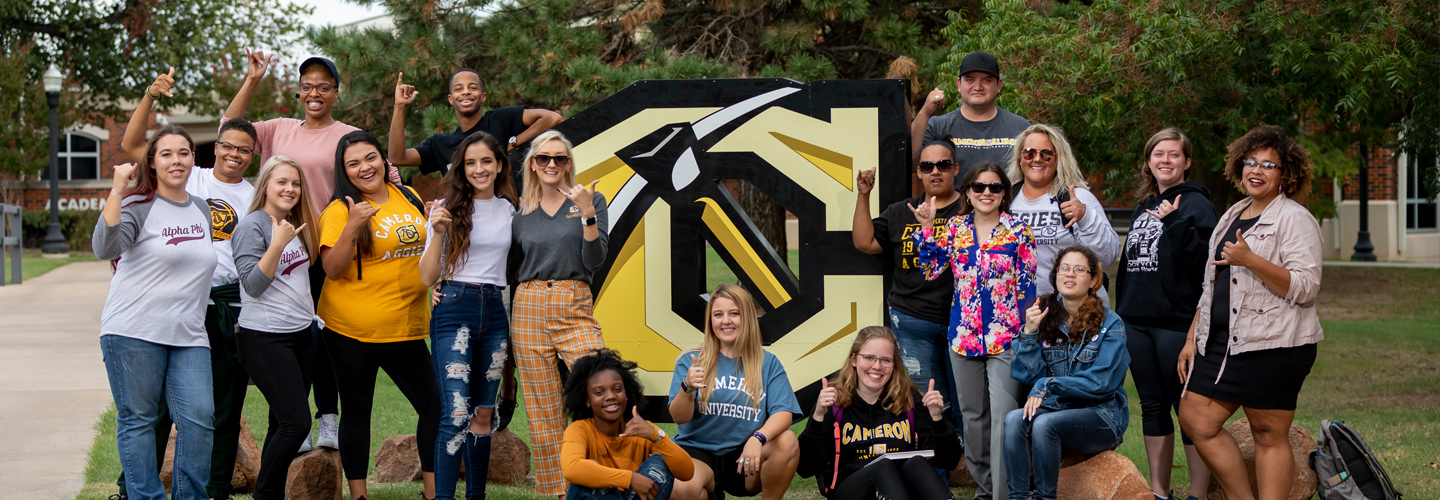# Assessment

## 150 Mathematics Curriculum Map

Courses

Students will demonstrate a conceptual understanding of algebra, geometry, trigonometry, and calculus concepts.

Students will apply major concepts from advanced algebra.

Students will analyze ordinary differential equations.

Students will construct mathematically rigorous and logically correct proofs.

Students will formulate mathematical models that arise in real-world situations.

Students will utilize technology as an effective tool in investigating, understanding, and applying mathematics.

Students will analyze and solve problems through the application of concepts from algebra, geometry, trigonometry, and calculus.

MATH 2215

Calc & Analytic Geom I

I

I

I

MATH 2235

Calc & Analytic Geom II

R

R

R

MATH 2244

Calc & Analytic Geom III

R

R

R

MATH 2613

Foundations of Math

I

MATH 3013

Intro Linear Algebra

I

R

MATH 3213

Abstract Algebra

I

R

MATH 3253

Differential Equations

R

M

M

R

MATH 4483

Intro To Real Analysis

R

R

MATH 4782

Mathematics Capstone I

M

M

M

M

MATH 4792

Mathematics Capstone II

M

M

M

M

MATH 1001

Technology for Mathematics

I

MATH 3001